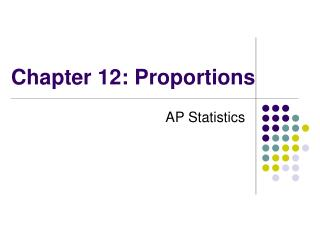DownloadDownload PresentationChapter 12: Proportions

# Chapter 12: Proportions

Télécharger la présentation## Chapter 12: Proportions

- - - - - - - - - - - - - - - - - - - - - - - - - - - E N D - - - - - - - - - - - - - - - - - - - - - - - - - - -
##### Presentation Transcript

1. Chapter 12: Proportions AP Statistics

2. Proportions • We use p for a population proportion. • p-hat is used for a sample proportion. • p-hat is defined as # of successes in sample # of observations in sample

3. Conditions for Inference on p • Data come from an SRS of the population • Population is at least 10x as large as sample • For hypothesis test: H0: p = p0, n is large enough that . • For a confidence interval

4. Confidence Interval for p • Draw an SRS of size n from a population with unknown proportion p of successes. An approximate level C confidence interval for p is: • Where z* is the upper (1 – C)/2 standard normal critical value.

5. Hypothesis Test • Draw an SRS of size n from a large population with unknown proportion p of successes. To test the hypothesis compute the z statistic

6. What proportion of M&M’s are blue? • Let’s create a 95% Confidence interval for the population proportion of blue M&M’s. • Collecting the data: Open your m&m’s and fill in the chart below for your package:

7. Set Up of the CI • Identify the population of interest and the parameter. Define Symbols. • Check conditions. • Mechanics • Interpretation in context

8. "ICFCI" format  ("I create fabulous confidence intervals") I: Introduce  A full sentence identifying the parameter in context and in symbol, eg, "I am creating a 99% confidence interval for p, the population proportion of blue m&m’s in 1.69 ounce bags.“ C: ConditionsCheck conditions as needed, including random sample, size n. F: FormulaWrite the entire formula with correct symbols.(df for t CI’s) C: CalculationsWrite in the values, including the z-or t-critical value. Then use calculator. I:  Interpret.Two sentences: one for the numbers in context ("I am 99% confident that the true proportion of blue m&m’s in 1.69 ounce bags lies in the interval...) and one for the method  (“The method produces an interval which captures the proportion 99% of time.")

9. Any Questions?

10. The Truth: • According to m&m’s these are the true proportions. Does your interval include 0.24?

11. Hypothesis Test • Orange m&m’s are most favorite, and it always seems like I don’t get enough of them. Let’s do a hypothesis test to determine whether the true proportion of orange m&m’s is 0.20 or if it different from 0.20.

12. Set up of the Test • Define symbols, check conditions. • State hypotheses in context. • Mechanics. • Conclusion in context.

13. Catch Backwards H: HypothesesState in symbols and in context C: ConditionsCheck conditions as needed, including random sample, n < .1N, evidence of normality if needed (np at least ten, etc., or NPP, or boxplot checked for symmetry, or n large, etc.) T: Test statisticWrite the entire formula with correct symbols, including df. Evaluate the test statistic by writing in the values and having the calculator produce the numbers (including, possibly, df's) A: Alpha Compare p-level to alpha, including sketch. C:  Conclude: Citing the comparison of p-level to alpha, state conclusion in context.

14. Choosing the sample size • The margin of error m, for a CI is: • Generally, we don’t know p*, so, you can either guess what you think p is, or use p* = .5 to be safe. • Solve for n, that’s the sample size.

15. Example • Barack Obama wants to know the proportion of voters in Ohio which prefer him over Hilary Clinton. Sampling costs money, but he wants to be accurate within 2%. What sample size would he need to achieve this. Assume p = 0.5. Use a 95% CI.

16. Exercises • p689: 12.5, p694: 12.7, 12.9,p697: 12.12, p698: 12.16